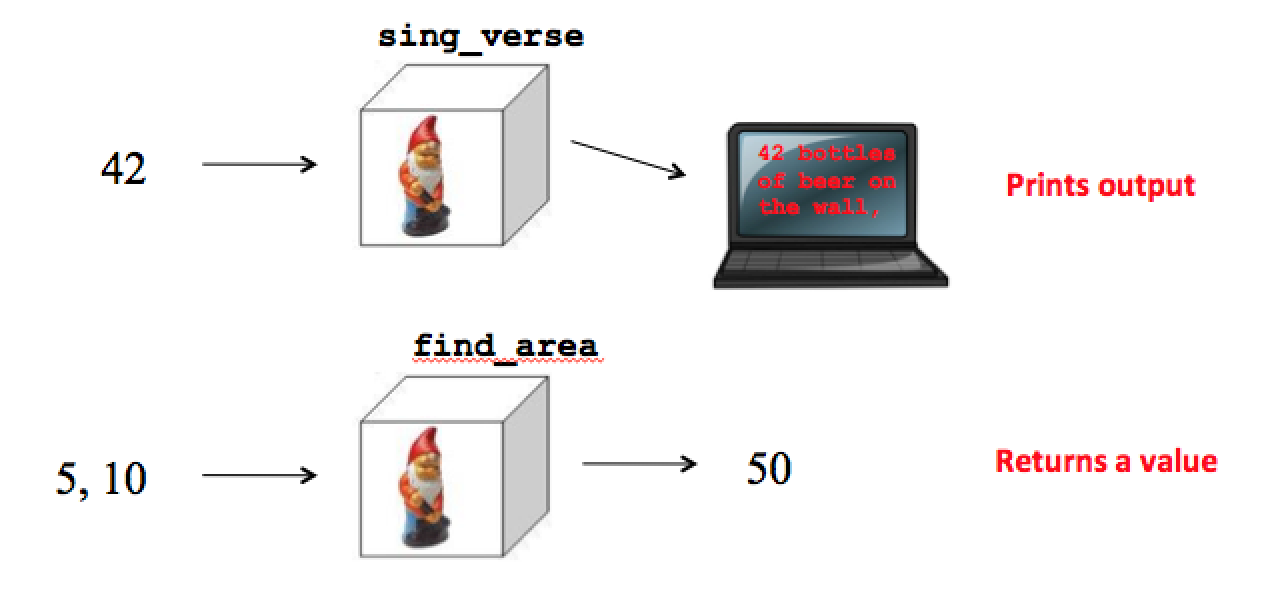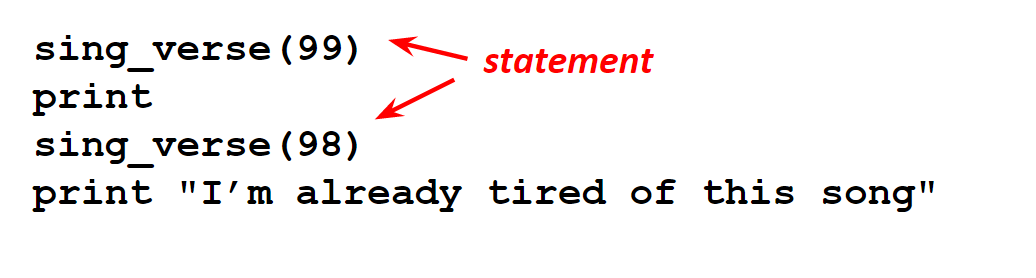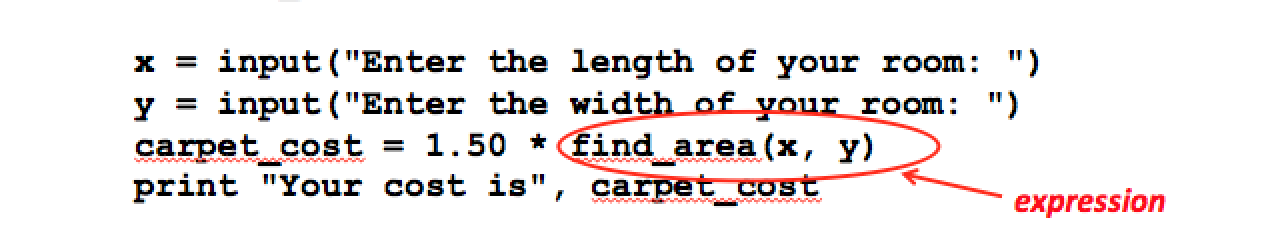# Chapter 8 - Value-returning functions¶

Last time we talked about the fact that when we define a function, we start by asking ourselves two questions:

• What are the parameters, that is, what pieces of information will this function need in order to do its job?
• Should the function produce its own output, or should it return a value?

So far we have only written functions that produce output. Now we are going to learn how to write value-returning functions.

## Value-returning functions¶

As an example to start with, remember that last time we wrote a function that computes the area of a rectangle, given the length and width.

Now, what if we don’t just want to print the area, but we want to use the value in another calculation? For example, suppose we are going to put carpet in a 10 by 12 foot room, and the carpet is \$1.50 per square foot. We could try writing a statement like this to determine the cost of the carpet:

carpet_cost = 1.50 * print_area(10, 12)

But something goes very wrong here. The function call print_area(10, 12) doesn’t actually give us the value of the area, it just prints the value on the screen! If we want to do something with the value other than printing it on the screen, we need to write a different kind of function. Instead of printing output, what we need is a return statement to give the function call a value.

Notice we have changed the name of the function from print_area to just “find_area”, since a call to the function no longer prints the area, it IS the area. The most important change is that we have replaced the print statement with a return statement, using the return keyword. The general form of a return statement is:

return expression

where expression is the value you want the function to have. It doesn’t have to be a single variable; it can be any expression. So we could also have written the find_area function without the temporary variable “result”:

Now it makes sense to write a statement like this:

carpet_cost = 1.50 * find_area(10, 12)

because find_area(10, 12) is just an expression with value 120.

Assuming that the area function is located in a file my_module.py, we could write an interactive script for determining the cost of carpeting a room:

## The special value None¶

You might wonder what happens if you try to use the value of a function that doesn’t return a value. For example, let’s try examining the value of a call to print_area:

The constant None is a special value in Python. It has the type “NoneType”. It’s the value you get from a function that doesn’t return a value using a return statement. Whenever you see None in your output, it means you are trying to print something that doesn’t have a value. Likewise, if you try to use the value None in a calculation, you’ll get an error message. Try this:

The output might be confusing at first, because it will print the area. This is because you called the print_area() function, which will print successfully. The the error is from the final line of the code, in computing carpet_cost.

TypeError: unsupported operand type(s): 'float' and 'NoneType'


The interpreter is telling us that it doesn’t know how to multiply a float with None.

## 1.3 Examples¶

Example 1. Write a function that takes one numeric argument, x, and returns the value of two times x plus one. This is a simple mathematical function that would be written in a math book as f(x) = 2x + 1. For lack of a better idea, we’ll just call it “f”. All that we need to do in the function body is double the parameter and add 1 and then return the result.

Example 2.

        sc-1-5: Rearrange the blocks to create a function that takes two strings and returns the longest one (you can just return the first one if they are the same length).def longest_string(first, second):
---
if len(second) > len(first):
---
longest = second
---
else:
---
longest = first
---
return longest

Example 3. Write a function that returns a letter grade of “A”, “B”, “C”, or “F” when given a score. This is the same logic we used previously for computing grades; the difference is that we want to put the code into a function and make the score a parameter.

The code you see below is not complete. What do you need to add to make sure all the conditions are met?

Example 4. Write a function that, given two integers x and y, determines whether x is divisible by y.

For this example, it makes sense that we will need two parameters representing the given values x and y. But what is the function supposed to return? Well, notice that a question such as, “Is 10 divisible by 3?” has an answer of either “True” or “False”. That is, the result of this function should be a boolean value. To check divisibility, we just have to look at the remainder. For example, we can see that 10 is not divisible by 3, because 10 divided by 3 leaves a remainder of 1. Try it out below. What will is_divisible(7/3) be? What will is_divisible(9,3) be? Here is a first attempt.

You might also notice that the whole function body could even be written on one line, without the temporary variable “result”:

We would read the assignment as, “result gets assigned the value of the expression x % y == 0”, which will either be the value True or the value False. This sort of thing looks strange at first, because we’re not used to using boolean values and boolean variables the same way we use numbers. But this kind of thing is incredibly useful in programming. In the next unit we’ll see some examples showing how such a function is used.

## 1.4 Two kinds of functions, and how we use them¶

In the last chapter we wrote functions that produce their own output. For example, our first function sing_verse, prints one verse of the song “99 bottles”. We would say that such a function has a “side-effect” because it actually does something that affects the world outside its “black box”. In this unit we wrote value-returning functions. For example, we wrote a function for computing the area of a rectangle, simply called find_area, that “returns” the area as the value of the function call. One way to think about these two different kinds of functions is to recall the distinction we made between expressions and statements:

An expression represents a value

A statement is an instruction to DO something

The two kinds of functions correspond to expressions and statements

A value-returning function such as find_area is used as an expression

A function that prints its own output such as sing_verse is used as a statementYou can see this if you compare how we used the two functions. Here is how we called the sing_verse function. We’re using each function call as a statement in this script.Compare this to the way we used the area function in the script we wrote above for finding the cost of carpeting a room. In this case, the function call is an expression we can use to figure out the total cost of the carpet.When you write a function, remember that you start by asking two questions.

1. What parameters does this function need? That is, “what information does this function need in order to do its job?”
2. Should this function produce its own output, or should it return a value?

In most cases, you’ll want a function to return a value, because such functions are the easiest to re-use. Here we wrote a user interface that uses the area function to compute the cost of carpet. It is easy to imagine using the area function for some other purpose, or in some other kind of user interface like a GUI. Since the area function does not produce its own output, we can easily re-use it in a different script. You might notice that all the built-in functions we have used, other than print and input, are value-returning functions.

A function like sing_verse really can’t be written as a value-returning function, because it’s job doesn’t involve the computation of a single value. A function like this is still useful because it helps us to organize our code into pieces that perform a particular task. Whenever we are faced with a large problem, we try to divide it into sub-problems that are easier to solve. In this example, printing all the verses to “99 bottles” is kind of a large problem, but printing just one verse is a small sub-problem. To print all the verses, we would just have to arrange to call sing_verse for each number between 99 and 0. As a peek ahead, we’ll see in a later chapter that we can do this very easily using something called a “for-loop”:

It might be hard to see the point of writing a function like find_area, when it is so easy to calculate the value directly as the length times the width. But really, it only seems that way because we are just starting out. We’re writing scripts and functions that are just a few lines long. Once they start to get even a little bit more complex, we’ll appreciate the fact that we can write the code once inside a function and re-use it multiple times. Modern software systems involve hundreds of thousands of lines of code, and without the ability to divide them in modular pieces like functions, it would literally be impossible for them to be written at all!